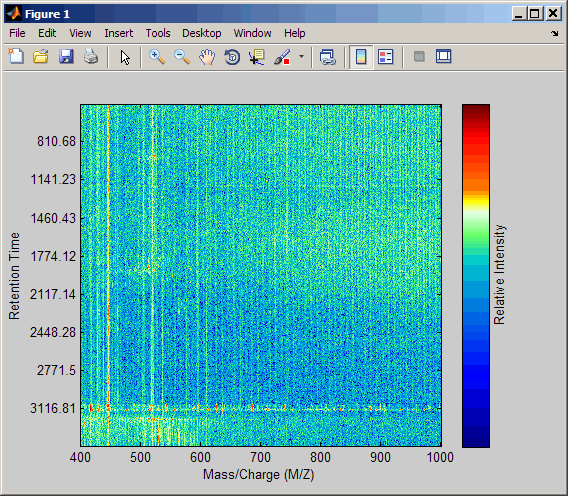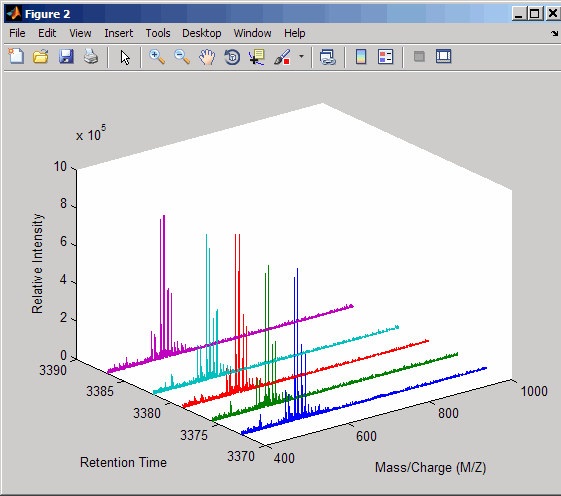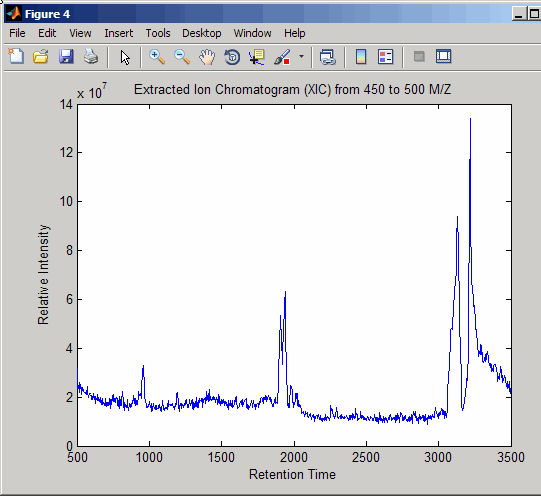# msppresample

Resample signal with peaks while preserving peaks

## Syntax

```[X, Intensities] = msppresample(Peaklist, N) msppresample(Peaklist, N, ...'Range', RangeValue, ...) msppresample(Peaklist, N, ...'FWHH', FWHHValue, ...) msppresample(Peaklist, N, ...'ShowPlot', ShowPlotValue, ...) ```

## Input Arguments

 `Peaklist` Either of the following:Two-column matrix, where the first column contains separation-unit values and the second column contains intensity values. The separation unit can quantify wavelength, frequency, distance, time, or m/z depending on the instrument that generates the signal data.Cell array of peak lists, where each element is a two-column matrix of separation-unit values and intensity values, and each element corresponds to a signal or retention time.TipYou can use the `mzxml2peaks` function or the `mspeaks` function to create the `Peaklist` matrix or cell array. `N` Integer specifying the number of equally spaced points (separation-unit values) in the resampled signal. `RangeValue` 1-by-2 vector specifying the minimum and maximum separation-unit values for the output matrix `Intensities`. `RangeValue` must be within `[min(inputSU) max(inputSU)]`, where `inputSU` is the concatenated separation-unit values from the input `Peaklist`. Default is the full range ```[min(inputSU) max(inputSU)]```. `FWHHValue` Value that specifies the full width at half height (FWHH) in separation units. The FWHH is used to convert each peak to a Gaussian shaped curve. Default is `median(diff(inputSU))/2`, where `inputSU` is the concatenated separation-unit values from the input `Peaklist`. The default is a rough approximation of resolution observed in the input data, `Peaklist`.TipTo ensure that the resolution of the peaks is preserved, set `FWHHValue` to half the distance between the two peaks of interest that are closest to each other. `ShowPlotValue` Controls the display of a plot of an original and resampled signal. Choices are `true`, `false`, or `I`, an integer specifying the index of a signal in `Intensities`. If you set to `true`, the first signal in `Intensities` is plotted. Default is:`false` — When return values are specified.`true` — When return values are not specified.

## Output Arguments

 `X` Vector of equally spaced, common separation-unit values for a set of signals with peaks. The number of elements in the vector equals `N`, or the number of rows in matrix `Intensities`. `Intensities` Matrix of reconstructed intensity values for a set of peaks that share the same separation-unit range. Each row corresponds to a separation-unit value, and each column corresponds to either a set of signals with peaks or a retention time. The number of rows equals `N`, or the number of elements in vector `X`.

## Description

Tip

Use the following syntaxes with data from any separation technique that produces signal data, such as spectroscopy, NMR, electrophoresis, chromatography, or mass spectrometry.

```[X, Intensities] = msppresample(Peaklist, N)``` resamples `Peaklist`, a peak list, by converting centroided peaks to a semicontinuous, raw signal that preserves peak information. The resampled signal has `N` equally spaced points. Output `X` is a vector of `N` elements specifying the equally spaced, common separation-unit values for the set of signals with peaks. Output `Intensities` is a matrix of reconstructed intensity values for a set of peaks that share the same separation-unit range. Each row corresponds to a separation-unit value, and each column corresponds to either a set of signals with peaks or a retention time. The number of rows equals `N`.

`msppresample` uses a Gaussian kernel to reconstruct the signal. The intensity at any given separation-unit value is taken from the maximum intensity of any contributing (overlapping) peaks.

Tip

`msppresample` is useful to prepare a set of signals for imaging functions such as `msheatmap` and preprocessing functions such as `msbackadj` and `msnorm`.

```msppresample(Peaklist, N, ... 'PropertyName', PropertyValue, ...)``` calls `msppresample` with optional properties that use property name/property value pairs. You can specify one or more properties in any order. Each `PropertyName` must be enclosed in single quotation marks and is case insensitive. These property name/property value pairs are as follows:

``` msppresample(Peaklist, N, ...'Range', RangeValue, ...)``` specifies a separation-unit range for the output matrix `Intensities` using the minimum and maximum separation values specified in the 1-by-2 vector `RangeValue`. `RangeValue` must be within `[min(inputSU) max(inputSU)]`, where `inputSU` is the concatenated separation-unit values from the input `Peaklist`. Default is the full range ```[min(inputSU) max(inputSU)]```

```msppresample(Peaklist, N, ...'FWHH', FWHHValue, ...)``` sets the full width at half height (FWHH) in separation units. The FWHH is used to convert each peak to a Gaussian shaped curve. Default is `median(diff(inputSU))/2`, where `inputSU` is the concatenated separation-unit values from the input `Peaklist`. The default is a rough approximation of resolution observed in the input data, `Peaklist`.

Tip

To ensure that the resolution of the peaks is preserved, set `FWHHValue` to half the distance between the two peaks of interest that are closest to each other.

```msppresample(Peaklist, N, ...'ShowPlot', ShowPlotValue, ...)``` controls the display of a plot of an original and resampled signal. Choices are `true`, `false`, or `I`, an integer specifying the index of a signal in `Intensities`. If you set to `true`, the first signal in `Intensities` is plotted. Default is:

• `false` — When return values are specified.

• `true` — When return values are not specified.

## Examples

1. Load a MAT-file, included with the Bioinformatics Toolbox™ software, that contains liquid chromatography/mass spectrometry (LC/MS) data variables. It includes `peaks`, a cell array of peak lists, where each element is a two-column matrix of m/z values and ion intensity values, and each element corresponds to a spectrum or retention time.

`load lcmsdata`
2. Resample the data, specifying 5000 m/z values in the resampled signal. Then create a heat map of the LC/MS data.

```[MZ,Y] = msppresample(ms_peaks,5000); msheatmap(MZ,ret_time,log(Y))```3. Plot the reconstructed profile spectra between two retention times.

```figure t1 = 3370; t2 = 3390; h = find(ret_time>t1 & ret_time<t2); [MZ,Y] = msppresample(ms_peaks(h),10000); plot3(repmat(MZ,1,numel(h)),repmat(ret_time(h)',10000,1),Y) xlabel('Mass/Charge (M/Z)') ylabel('Retention Time') zlabel('Relative Intensity')```4. Resample the data to plot the Total Ion Chromatogram (TIC).

```figure [MZ,Y] = msppresample(ms_peaks,5000); plot(ret_time,sum(Y)) title('Total Ion Chromatogram (TIC)') xlabel('Retention Time') ylabel('Relative Intensity')```5. Resample the data to plot the Extracted Ion Chromatogram (XIC) in the 450 to 500 m/z range.

```figure [MZ,Y] = msppresample(ms_peaks,5000,'Range',[450 500]); plot(ret_time,sum(Y)) title('Extracted Ion Chromatogram (XIC) from 450 to 500 M/Z') xlabel('Retention Time') ylabel('Relative Intensity')```## Version History

Introduced in R2007a